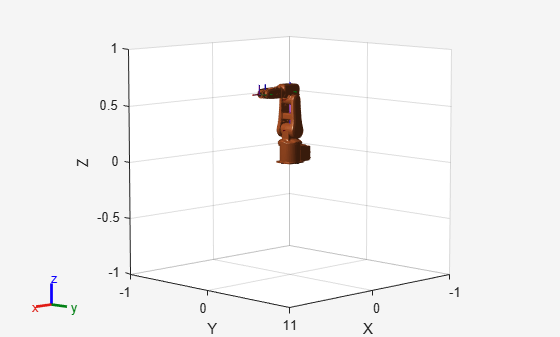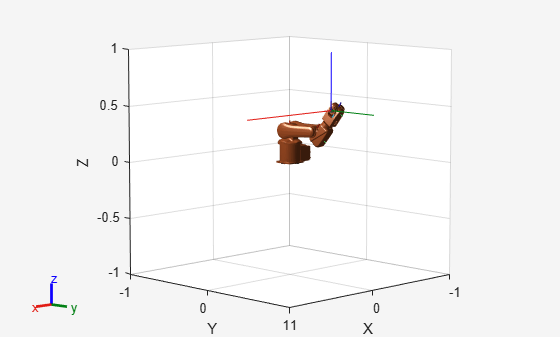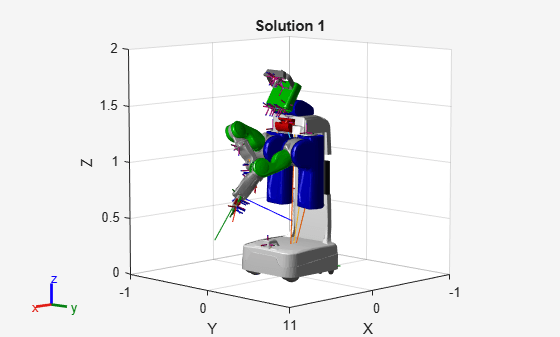# generateIKFunction

Generate function for closed-form inverse kinematics

Since R2020b

## Syntax

``ikFunction = generateIKFunction(analyticalIK,functionName)``

## Description

example

````ikFunction = generateIKFunction(analyticalIK,functionName)` generates a function with a specified name, `functionName`, that computes the closed-form solutions for inverse kinematics (IK) for a selected kinematic group to achieve a desired end-effector pose. `generateIKFunction` also writes a function to the current directory as a file with the specified function name.To generate a list of configurations that achieve the desired end-effector pose, use the generated function `ikFunction`. The specified ``` analyticalInverseKinematics``` object `analyticalIK` must contain a valid kinematic group. For information on determining valid kinematic groups, see the `showdetails` function.For the syntax of the generated function, see the `ikFunction` output argument.```

## Examples

collapse all

Generate closed-form inverse kinematics (IK) solutions for a desired end effector. Load the provided robot model and inspect details about the feasible kinematic groups of base and end-effector bodies. Generate a function for your desired kinematic group. Inspect the various configurations for a specific end-effector pose.

Robot Model

Load the ABB IRB 120 robot model into the workspace. Display the model.

```robot = loadrobot('abbIrb120','DataFormat','row'); show(robot);```Analytical IK

Create the analytical IK solver. Show details for the robot model, which lists the different kinematic groups available for closed-form analytical IK solutions. Select the second kinematic group by clicking the Use this kinematic group link in the second row of the table.

```aik = analyticalInverseKinematics(robot); showdetails(aik)```
```-------------------- Robot: (8 bodies) Index Base Name EE Body Name Type Actions ----- --------- ------------ ---- ------- 1 base_link link_6 RRRSSS Use this kinematic group 2 base_link tool0 RRRSSS Use this kinematic group ```

Inspect the kinematic group, which lists the base and end-effector body names. For this robot, the group uses the '`base_link'` and `'tool0'` bodies, respectively.

`aik.KinematicGroup`
```ans = struct with fields: BaseName: 'base_link' EndEffectorBodyName: 'tool0' ```

Generate Function

Generate the IK function for the selected kinematic group. Specify a name for the function, which is generated and saved in the current directory.

`generateIKFunction(aik,'robotIK');`

Specify a desired end-effector position. Convert the xyz-position to a homogeneous transformation.

```eePosition = [0 0.5 0.5]; eePose = trvec2tform(eePosition); hold on plotTransforms(eePosition,tform2quat(eePose)) hold off```Generate Configuration for IK Solution

Specify the homogeneous transformation to the generated IK function, which generates all solutions for the desired end-effector pose. Display the first generated configuration to verify that the desired pose has been achieved.

```ikConfig = robotIK(eePose); % Uses the generated file show(robot,ikConfig(1,:)); hold on plotTransforms(eePosition,tform2quat(eePose)) hold off```Display all of the closed-form IK solutions sequentially.

```figure; numSolutions = size(ikConfig,1); for i = 1:size(ikConfig,1) subplot(1,numSolutions,i) show(robot,ikConfig(i,:)); end```Some manipulator robot models have large degrees-of-freedom (DOFs). To reach certain end-effector poses, however, only six DOFs are required. Use the `analyticalInverseKinematics` object, which supports six-DOF robots, to determine various valid kinematic groups for this large-DOF robot model. Use the `showdetails` object function to get information about the model.

Load Robot Model and Generate IK Solver

Load the robot model into the workspace, and create an `analyicalInverseKinematics` object. Use the `showdetails` object function to see the supported kinematic groups.

```robot = loadrobot('willowgaragePR2','DataFormat','row'); aik = analyticalInverseKinematics(robot); opts = showdetails(aik);```
```-------------------- Robot: (94 bodies) Index Base Name EE Body Name Type Actions ----- --------- ------------ ---- ------- 1 l_shoulder_pan_link l_wrist_roll_link RSSSSS Use this kinematic group 2 r_shoulder_pan_link r_wrist_roll_link RSSSSS Use this kinematic group 3 l_shoulder_pan_link l_gripper_palm_link RSSSSS Use this kinematic group 4 r_shoulder_pan_link r_gripper_palm_link RSSSSS Use this kinematic group 5 l_shoulder_pan_link l_gripper_led_frame RSSSSS Use this kinematic group 6 l_shoulder_pan_link l_gripper_motor_accelerometer_link RSSSSS Use this kinematic group 7 l_shoulder_pan_link l_gripper_tool_frame RSSSSS Use this kinematic group 8 r_shoulder_pan_link r_gripper_led_frame RSSSSS Use this kinematic group 9 r_shoulder_pan_link r_gripper_motor_accelerometer_link RSSSSS Use this kinematic group 10 r_shoulder_pan_link r_gripper_tool_frame RSSSSS Use this kinematic group ```

Select a group programmatically using the output of the `showdetails` object function, `opts`. The selected group uses the left shoulder as the base with the left wrist as the end effector.

```aik.KinematicGroup = opts(1).KinematicGroup; disp(aik.KinematicGroup)```
``` BaseName: 'l_shoulder_pan_link' EndEffectorBodyName: 'l_wrist_roll_link' ```

Generate the IK function for the selected group.

`generateIKFunction(aik,'willowRobotIK');`

Solve Analytical IK

Define a target end-effector pose using a randomly-generated configuration.

```rng(0); expConfig = randomConfiguration(robot); eeBodyName = aik.KinematicGroup.EndEffectorBodyName; baseName = aik.KinematicGroup.BaseName; expEEPose = getTransform(robot,expConfig,eeBodyName,baseName);```

Solve for all robot configurations that achieve the defined end-effector pose using the generated IK function. To ignore joint limits, specify `false` as the second input argument.

`ikConfig = willowRobotIK(expEEPose,false);`

To display the target end-effector pose in the world frame, get the transformation from the base of the robot model, rather than the base of the kinematic group. Display all of the generated IK solutions by specifying the indices for your kinematic group IK solution in the configuration vector used with the `show` function.

```eeWorldPose = getTransform(robot,expConfig,eeBodyName); generatedConfig = repmat(expConfig, size(ikConfig,1), 1); generatedConfig(:,aik.KinematicGroupConfigIdx) = ikConfig; for i = 1:size(ikConfig,1) figure; ax = show(robot,generatedConfig(i,:)); hold all; plotTransforms(tform2trvec(eeWorldPose),tform2quat(eeWorldPose),'Parent',ax); title(['Solution ' num2str(i)]); end```## Input Arguments

collapse all

Analytical inverse kinematics solver, specified as an `analyticalInverseKinematics` object.

Name of the generated function, specified as a string scalar or character vector.

Example: `"jacoIKSolver"`

Data Types: `char` | `string`

## Output Arguments

collapse all

IK solver for the selected kinematic group, returned as a function handle. The function generates closed-form solutions and has these syntax options:

```robotConfig = ikFunction(eeTransform) robotConfig = ikFunction(eeTransform,enforceJointLimits) robotConfig = ikFunction(eeTransform,enforceJointLimits,sortByDistance) robotConfig = ikFunction(eeTransform,enforceJointLimits,sortByDistance,referenceConfig) ```

Desired end-effector pose, specified as a 4-by-4 homogeneous transformation matrix. To generate a transformation matrix from an xyz-position and quaternion orientation, use the `trvec2tform` and `quat2tform` functions on the respective coordinates and multiply the resulting matrices.

```tform1 = trvec2tform([x y z]); tform2 = quat2tform([qw qx qy qz]); eeTransform = tform1*tform2;```

Data Types: `single` | `double`

Enforce joint limits of the rigid body tree model, specified as a logical, `1` (`true` or `0` (`false`). When set to `false`, the solver ignores the joint limits of the robot model in the RigidBodyTree property of the `analyticalInverseKinematics` object.

Data Types: `logical`

Sort configurations based on distance from desired pose, specified as a logical, `1` (`true` or `0` (`false`).

Data Types: `logical`

Reference configuration for the IK solution, specified as an n-element vector, where n is the number of nonfixed joints in the rigid body tree robot model. Each element corresponds to a joint position as either a rotation angle in radians for revolute joints or a linear distance in meters for prismatic joints.

Data Types: `single` | `double`

## Version History

Introduced in R2020b